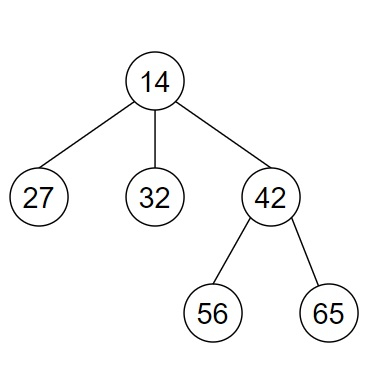# Program to find the root of a n-ary tree in Python

Suppose, we are given the nodes of an n-ary tree in an array. We have to find and return the root node of the tree by reconstructing it. The full tree has to be displayed from the returned node in preorder notation.

So, if the input is likethen the output will be

[14, 27, 32, 42, 56, 65]

We will use the root of the tree to display the pre order traversal of the tree. So, the output is a pre order traversal of the tree.

To solve this, we will follow these steps −

• indegree := a new map containing integer values

• for each node in tree, do

• for each child in children pointers of the node, do

• indegree[value of child] := indegree[value of child] + 1

• for each node in tree, do

• if indegree[value of node] is same as 0, then

• return node

• return null

## Example (Python)

Let us see the following implementation to get better understanding −

Live Demo

import collections
class Node:
def __init__(self, value, child = None) -> None:
self.val = value
self.children = []
if child != None:
for value in child:
self.children.append(value)

def solve(tree):
indegree = collections.defaultdict(int)
for node in tree:
for child in node.children:
indegree[child.val] += 1
for node in tree:
if indegree[node.val] == 0:
return node
return None

def treeprint(node, tree):
if node == None:
tree.append("None")
return tree
if tree == None:
tree = []
tree.append(node.val)
for child in node.children:
treeprint(child, tree)
return tree

node6 = Node(65)
node5 = Node(56)
node4 = Node(42, [node5, node6])
node3 = Node(32)
node2 = Node(27)
node1 = Node(14, [node2, node3, node4])
tree = [node2, node1, node5, node3, node6, node4]

root = solve(tree)
print(treeprint(root, None))

## Input

node6 = Node(65)
node5 = Node(56)
node4 = Node(42, [node5, node6])
node3 = Node(32)
node2 = Node(27)
node1 = Node(14, [node2, node3, node4])
tree = [node2, node1, node5, node3, node6, node4]

## Output

[14, 27, 32, 42, 56, 65]